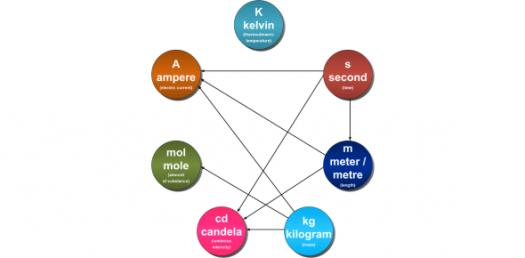10 Questions | Total Attempts: 1218Settings.

• 1.
The SI unit for temperature is in ........
• A.

Degree Celsius

• B.

Kelvin

• C.

Newton

• D.

Joules

• 2.
The SI unit for length is in ......
• A.

Metre

• B.

Centimetre

• C.

Kilometre

• D.

Decimetre

• 3.
The SI unit for time is in ........
• A.

Minute

• B.

Second

• C.

Hour

• D.

Hertz

• 4.
The SI unit for current is in ........
• A.

A

• B.

C

• C.

V

• D.

J

• 5.
The SI unit for mass is in ....
• A.

Kilogram

• B.

Gram

• C.

Tonnes

• D.

Milligram

• 6.
The SI unit for length is in ......
• A.

M

• B.

Cm

• C.

Km

• D.

Dm

• 7.
The SI unit for mass is in ....
• A.

Kg

• B.

G

• C.

Mg

• D.

Ng

• 8.
The SI unit for time is in ........
• A.

Min

• B.

S

• C.

Hr

• D.

Hz

• 9.
The SI unit for temperature is in ........
• A.

°C

• B.

K

• C.

N

• D.

J

• 10.
The SI unit for current is in ........
• A.

Ampere

• B.

Coulomb

• C.

Volts

• D.

Joules

Related TopicsBack to top